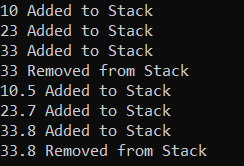# Template in C++

## Template in C++ with Examples

In this article, I am going to discuss Template in C++ with Examples. Please read our previous article where we discussed Exception Handling in C++ with Examples. This is one of the most important topics in C++. Let us understand this with some examples.

##### Template in C++

Template in C++ allows us to define Generic Functions and Generic Classes. That means using a Template we can implement Generic Programming in C++. Generic Programming is a technique where generic types are used as parameters and hence, they are going to work with a variety of data types. Template in C# can be represented in two ways. They are as follows.

1. Function templates
2. Class templates
##### Template Functions in C++

Templates are used for generic programming. The generalization is based on the data type. So, we can write a code that will work for any type of data. We have already shown you how to work with functions. Let us understand the function template with an example. Please have a look at the below code.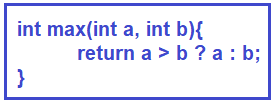The above code will find out the maximum of two integers. Suppose, we want to make this work for any type of data, then we can say,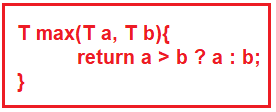Here instead of writing int, we have written T, so that this function can work for any data type: float, int, double, etc. So, this function now can work for any data type. So, we can pass any primitive type or any user-defined class or structure.

If you want your own classes to be passed and you want this function to find a maximum of two, then in your class you must override the ‘>’ operator that is the greater than operator. You should provide the functionality to know which object is greater. Then this function will work for your classes also.

##### Example to Understand Template Functions in C++:

Let us take an example of a function where we can pass multiple types of arguments.

template<class T, class R>
cout << x + y;
}

Here we have Add function which takes T type variable x and R type variable y. This function will print the sum of x and y. Now we are calling this function by passing one value as an integer and another value as a double,

After this call, T will become integer type and R will become double type. So, we can even have multiple parameters in the template. If required, you can use multiple data types also. For a better understanding, please have a look at the below example.

```#include <iostream>
using namespace std;
template <class T, class R>
void Add (T x, R y)
{
cout << x + y << endl;
}

int main()
{
//Integer and Integer
//Float and Float
//Integer and double
//Float and Integer
return 0;
}
```
###### Output: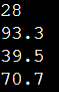##### Template Classes in C++

Now, let us understand template classes with some examples. Please have a look at the following code. Now let us look at the template class. So, for that, we have taken an example class Stack. I guess you already know about the stack data structures. So, we are writing a class for stacks and implementing a stack using an array. For implementing a stack, we have taken an array that is an array of size n whose name is S, and also have a Top pointer to point at a particular index. So, initially, we know that the top pointer will be at the -1 index. Then we have two functions for pushing a value into the stack and popping the value from the stack.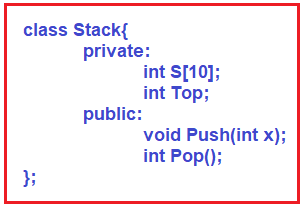If you look closely, you’ll notice that this is a Stack that only holds integers values. This will not work for the float type of data, a character type of data, or a string type of data. Now the problem is if we want a floating stack, then we should write another class, and if you want a string stack, then we also need to have a separate class.

So, instead of writing many classes for different data types, we can write a single class for all data types. The question is, how? The answer is, by making the class a template. Let us see how to make the above class as a template. Please have a look at the following code.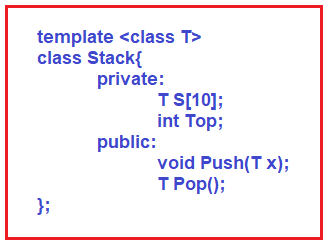As you can see in the above code, in the beginning, we have defined a class T, which is of type template. Then we modified the data type of the array from int type to template T and the Top pointer remains the same because it is pointing at the index. So, the index is always an integer. Then we modify the Push function parameter from the int type to T. Because push will insert the data of type T. Then the Pop function, which pops out the value and returns that value. So, what type of value should it return? It depends on the type of stack. So, we have modified the Pop function’s return type to T. Then we can implement the Push and Pop function as follows.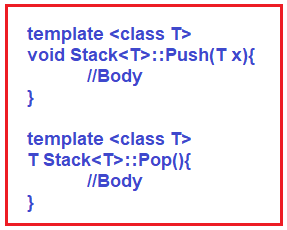So, in this way, we can implement the Pop and Push functions. Inside these functions, you can write your own code. We have just shown you the syntax for the template data type.

By making a class as a template class, we can use that class for multiple primitive data types and the same is valid for template functions. And when we implement the functions outside the class using the scope resolution operator, we must write a template.

Now one more thing, when we create an object of the Stack class, we have to give a primitive data type to create the object. For example,
Stack<int> S1;
Stack<float> S2;
Stack<double> S3;

So, this is all about Templates in C++. The template is the most powerful feature in C++ Language. This feature will reduce the work of programming which makes programming easy. Now let us look at the complete program.

##### Example to Understand Template Functions and Classes in C++
```#include <iostream>
using namespace std;

template <class T>
class Stack
{
private:
T * stk;
int top;
int size;
public:
Stack (int sz)
{
size = sz;
top = -1;
stk = new T[size];
}
void Push(T x);
T Pop();
};

template <class T>
void Stack<T>::Push(T x)
{
if (top == size - 1)
cout << "Stack is Full" << endl;
else
{
top++;
stk[top] = x;
cout << x <<" Added to Stack" << endl;
}
}

template<class T>
T Stack<T>::Pop()
{
T x = 0;
if (top == -1)
cout << "Stack is Empty" << endl;
else
{
x = stk[top];
top--;
cout << x <<" Removed from Stack" << endl;
}
return x;
}

int main()
{
//Stack of Integer
Stack<float> stack1(10);
stack1.Push(10);
stack1.Push(23);
stack1.Push(33);
stack1.Pop();

//Stack of double
Stack<double> stack2(10);
stack2.Push(10.5);
stack2.Push(23.7);
stack2.Push(33.8);
stack2.Pop();

return 0;
}
```
###### Output: